Homework Help Question & Answers

# A student is running a titration in the laboratory to determine the alkalinity (Total, bicarbonate and...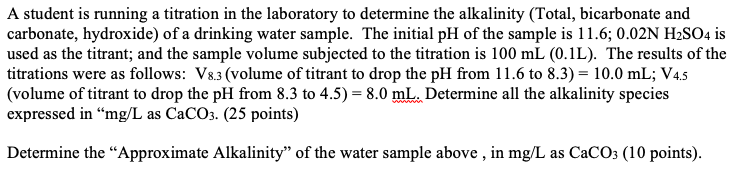A student is running a titration in the laboratory to determine the alkalinity (Total, bicarbonate and carbonate, hydroxide) of a drinking water sample. The initial pH of the sample is 11.6; 0.02N H2SO4 is used as the titrant; and the sample volume subjected to the titration is 100 mL (0.1L). The results of the titrations were as follows: 18.3 (volume of titrant to drop the pH from 11.6 to 8.3) = 10.0 mL; V4.5 (volume of titrant to drop the pH from 8.3 to 4.5) = 8.0 mL. Determine all the alkalinity species expressed in "mg/L as CaCO3. (25 points) Determine the "Approximate Alkalinity" of the water sample above , in mg/L as CaCO3 (10 points).

#### Homework Answers

Answer #1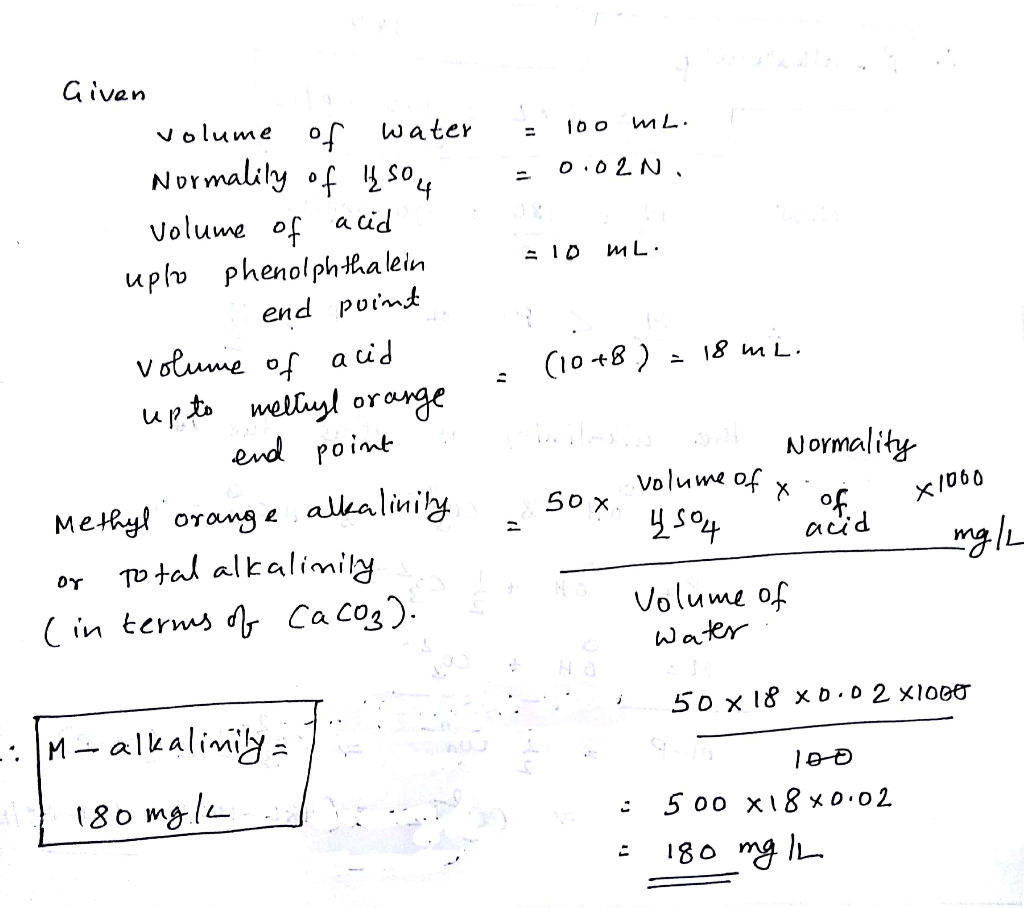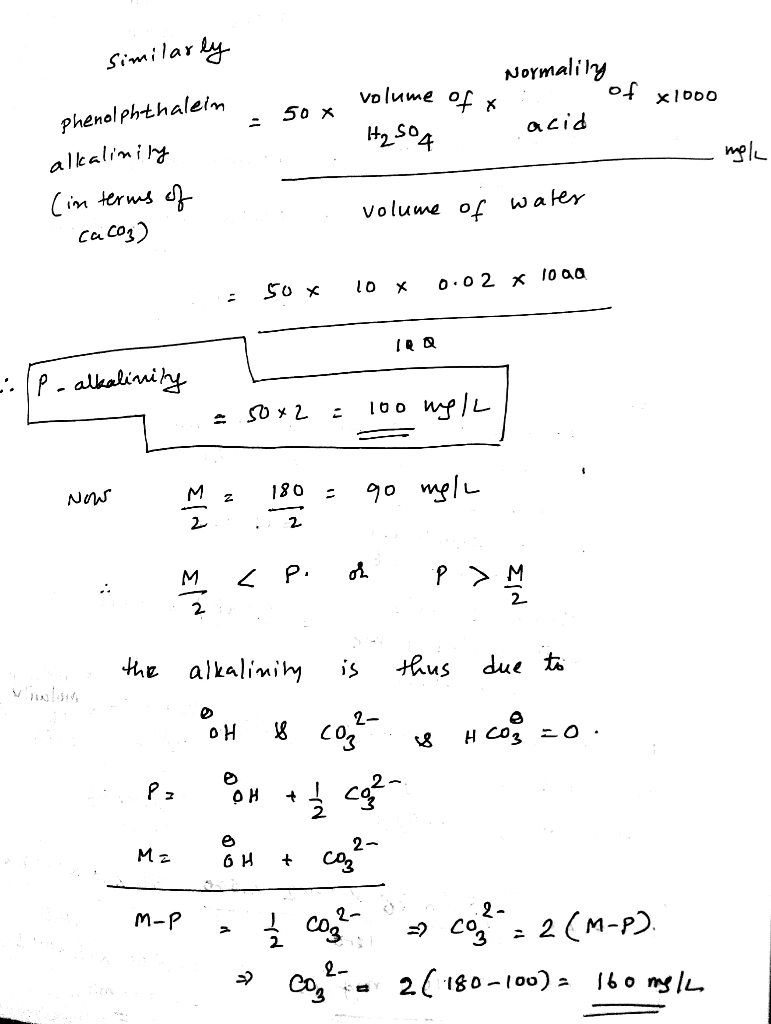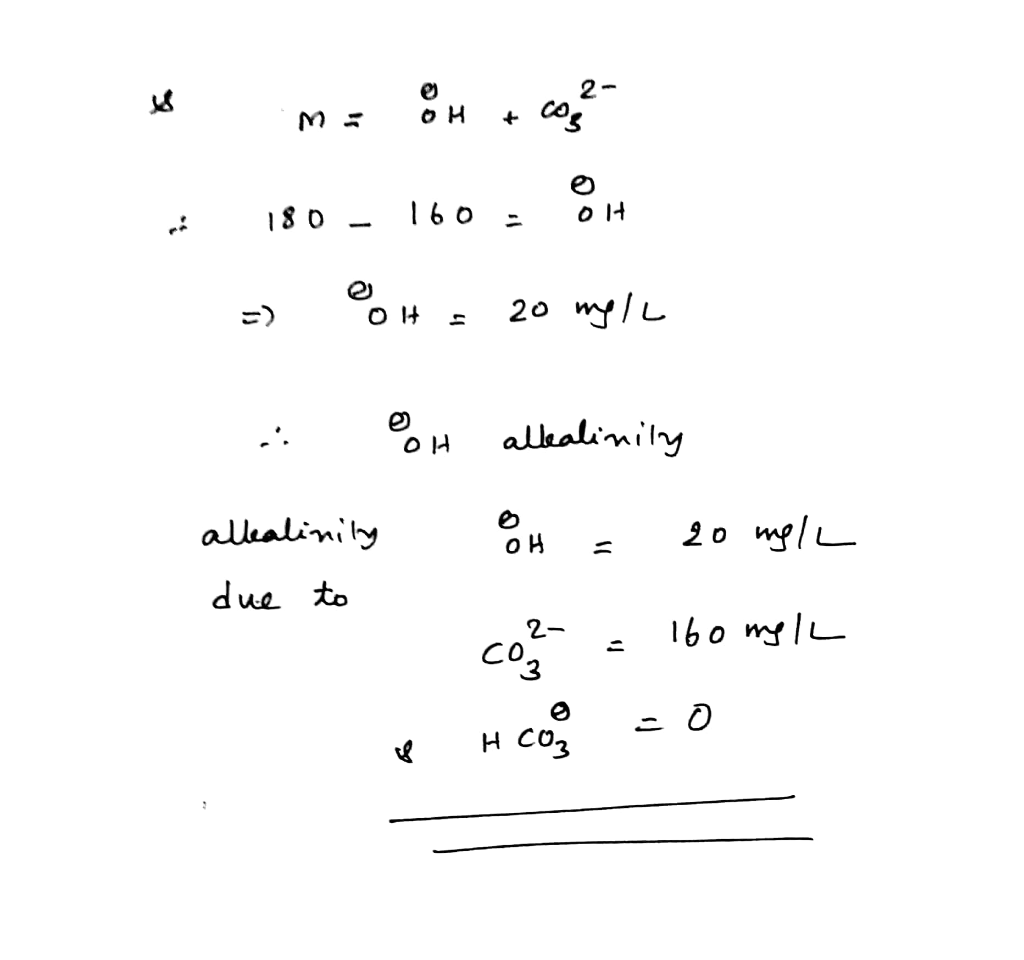Know the answer?
Your Answer:

#### Post as a guest

Your Name:

What's your source?

#### Earn Coin

Coins can be redeemed for fabulous gifts.

Not the answer you're looking for? Ask your own homework help question. Our experts will answer your question WITHIN MINUTES for Free.
Similar Homework Help Questions
• ### Alkalinity

The following properties of a water sample are measured: pH = 10.24, alkalinityfrom carbonate of 120 mg/L CaCO3. What is the quantity of alkalinity (in mg/L CaCO3) caused by the bicarbonate ion? The alkalinity is only due to inorganic species ofC and OH-.

• ### In an alkalinity titration of a 100.0-mL sample of water from a hot spring, 2.56 mL...

In an alkalinity titration of a 100.0-mL sample of water from a hot spring, 2.56 mL of a 0.0355 M solution of HCl is needed to reach the first equivalence point (pH=8.3) and another 10.42 mL is needed to reach the second equivalence point (pH=4.0). If the alkalinity of the spring water is due only to the presence of carbonate and bicarbonate, what are the concentrations of each of them?

• ### need HW help thx! 2. For the following, assume pKi = 6.35 and pK2 10.33. (a) The alkalinity and initial pH of a wate...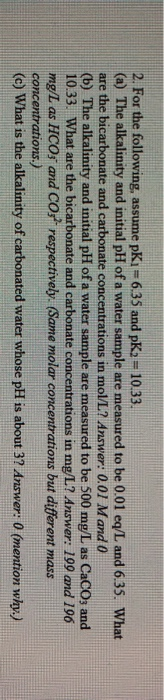need HW help thx! 2. For the following, assume pKi = 6.35 and pK2 10.33. (a) The alkalinity and initial pH of a water sample are measured to be 0.01 eq/L and 6.35. What are the bicarbonate and carbonate concentrations in mol/L? Answer: 0.01 Mand 0 (b) The alkalinity and initial pH of a water sample are measured to be 500 mg/L as CaCO3 and 10.33. What are the bicarbonate and carbonate concentrations in mg/L? Answer: 199 and 196 mg/L...

• ### Determine the alkalinity in mg/L CaCOs for DI water and tap water based on the number of digits (...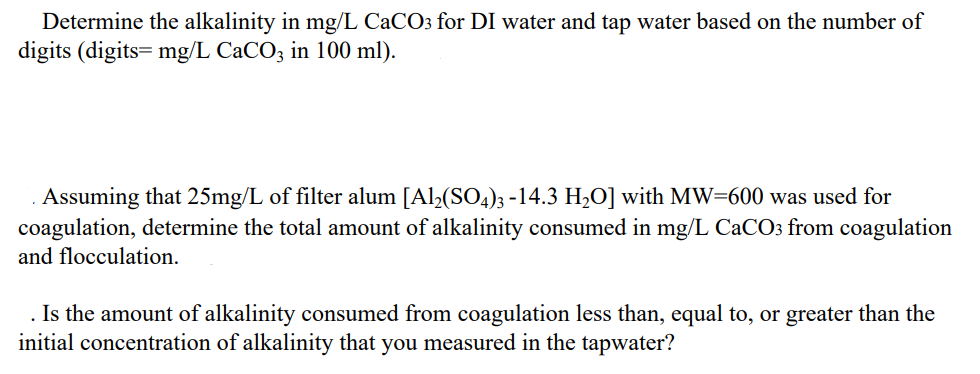To pH of 8.3 To pH of 4.5 0 320 Alkalinity. Wastewater Problem. Determine the alkalinity in mg/L CaCOs for DI water and tap water based on the number of digits (digits= mg/L CaCO3 in 100 ml) Assuming that 25mg/L of filter alum [Al2(SO4)3-14.3 H2O] with MW-600 was used for coagulation, determine the total amount of alkalinity consumed in mg/L CaCOs from coagulation and flocculation. Is the amount of alkalinity consumed from coagulation less than, equal to, or greater than...

• ### 11. Calculate for the following samples of water the hydroxide, carbonate and bicarbonate alkalinity by b)...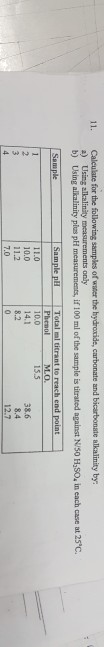11. Calculate for the following samples of water the hydroxide, carbonate and bicarbonate alkalinity by b) Using alkalinity plas pH measurements, if 100 ml of the sample s titrated against Niso H,so, in each case at 250 11.0 10.0 11.2 7.0 10.0 14.1 38.6

• ### please help with this question. Extra credit Homework on Acid-Base Titration You can use the technique...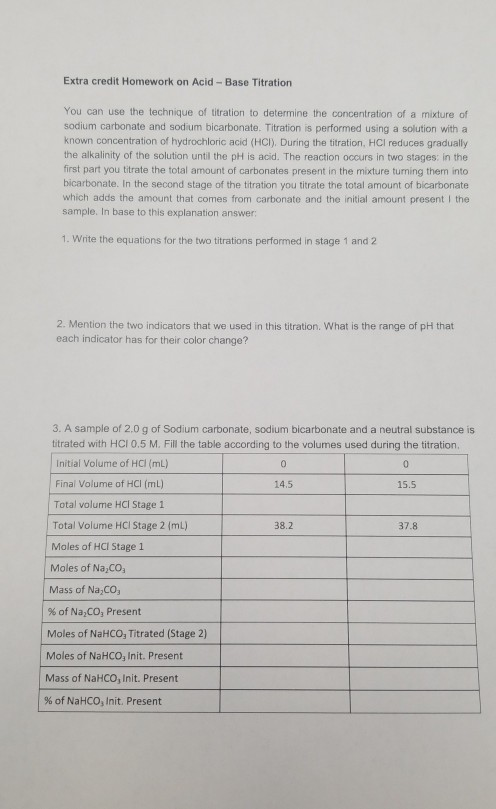please help with this question. Extra credit Homework on Acid-Base Titration You can use the technique of titration to determine the concentration of a mixture of sodium carbonate and sodium bicarbonate. Titration is performed using a solution with a known concentration of hydrochloric acid (HCI). During the titration, HCl reduces gradually the alkalinity of the solution until the pH is acid. The reaction occurs in two stages in the first part you titrate the total amount of carbonates present in...

• ### Can you please solve this? A 1.0000 g sample that contains both sodium carbonate and sodium...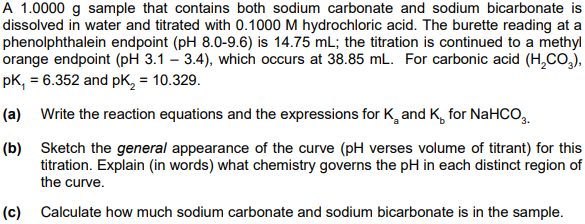Can you please solve this? A 1.0000 g sample that contains both sodium carbonate and sodium bicarbonate is dissolved in water and titrated with 0.1000 M hydrochloric acid. The burette reading at a phenolphthalein endpoint (pH 8.0-9.6) is 14.75 mL; the titration is continued to a methyl orange endpoint (pH 3.1 – 3.4), which occurs at 38.85 mL. For carbonic acid (H,CO), pK, = 6.352 and pk, = 10.329. (a) Write the reaction equations and the expressions for K and...

• ### Using the chemical analysis of surface water determine alkalinity, hardness, and total dissolved...

A chemical analysis of a surface water yields the following data:Ion Concentration mg/LCa2+90Mg2+30Na+72K+6Cl-120SO42-225HCO3-165pH7.5A.) Determine the alkalinity expressed as CaCO3B.)Determine the hardness as CaCO3C.) Estimate the total dissolved solids

• ### test for common ions are run on a sample of water Fall Semester, 2018 Tutorial 4...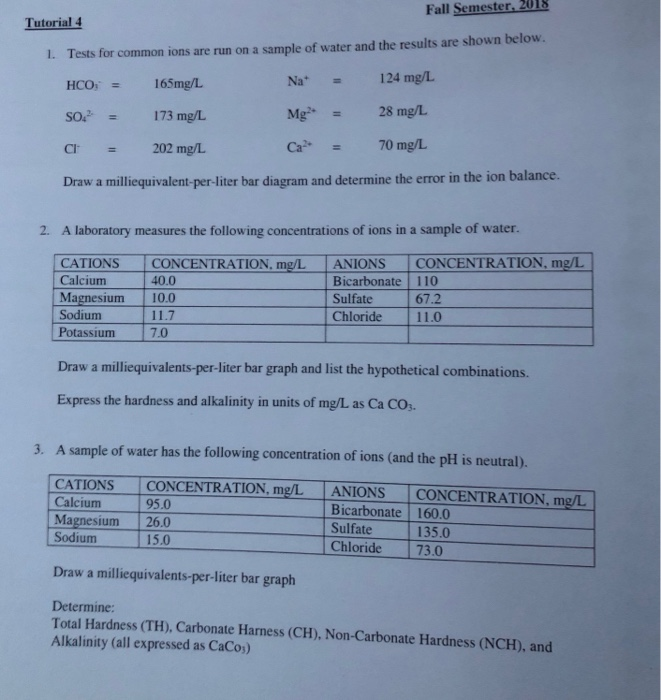test for common ions are run on a sample of water Fall Semester, 2018 Tutorial 4 Tests for common ions are run on a sample of water and the results are shown below HCO, 165mg/L so'. 173 mg/L cr = 202 mg/L Draw a milliequivalent-per-iter bar diagram and determine the error in the ion balance. L. Na+ Mg> Ca2+ 124 mg/L 28 mg/L 70 mg/L = = 2. A laboratory measures the following concentrations of ions in a sample of...

• ### CHomework Chapter 17 Plotting a Titration Curve for a Carbonate-Bicarbonate System 9 of 24> Carbo...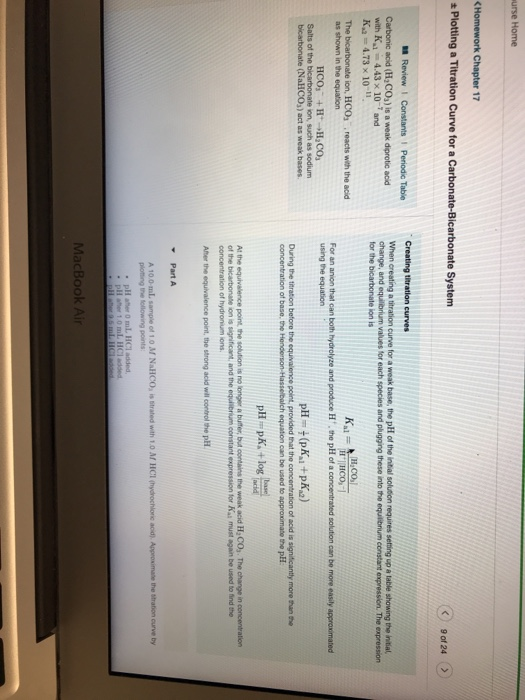CHomework Chapter 17 Plotting a Titration Curve for a Carbonate-Bicarbonate System 9 of 24> Carbonic acid (H2COa) is a weak diprotic acid with K4.43 x 10-7 and K 4.73 x 10-11 change, and equilibrium values for each species and plugging these into the equlibrium constant expression. The expression HCO as shown in the equation using the equation Salts of the bicarbonate ion, such as sodium During the titration before the equivalence point, provided that the concentration of acid is significantly...